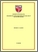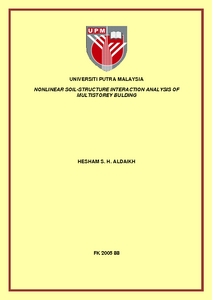# Nonlinear soil-structure interaction analysis of multistorey building

## Citation

S. H. Aldaikh, Hesham (2005) Nonlinear soil-structure interaction analysis of multistorey building. Masters thesis, Universiti Putra Malaysia.

## Abstract

The interaction among structures, their foundations and the soil medium below the foundations alter the actual behaviour of the structure considerably than what is obtained from the consideration of the structure alone. Conventionally, superstructures are usually analyzed by assuming the structure to be fixed at the foundation level. Such an analysis neglects the flexibility of foundation and compressibility of soil mass. It is also assumed, conventionally, that the soil is behaving linearly neglecting that fact that it is nonlinear in nature. In this study an attempt has been made to carry out a two dimensional linear and nonlinear analysis of the problem of a multistory building incorporating soil-structure interaction with respect to nonhomogenous soil properties in Malaysia. Two techniques of analysis have been carried out, in the first, linear stress strain relationship is assumed for the soil where finite element method has been employed in modelling the superstructure members and foundation beam while Winkler’s springs have been attached to the foundation beam to represent the soil layer below foundation, and then a linear coupled finite infinite element modelling is done. Three noded isoparametric beam bending element with three degrees of freedom that takes into account of the transverse shear forces and axial flexural interaction, this element is used to represent the frame members in all types of analyses. Eight noded isoparametric quadrilateral finite element is used to represent the near filed of soil while the far field is represented by using five noded isoparametric infinite element. In the second analysis, the same coupled finite-infinite element modelling is used, the that, the soil is considered to behave nonlinear and a hyperbolic model is used to take this nonlinearity into account. The result showed the importance of taking soil structure interaction into account,results obtained from each analysis have been obtained and comparison among various analyses has been stated.Preview
PDF
FK 2005 88RR.pdfView Item